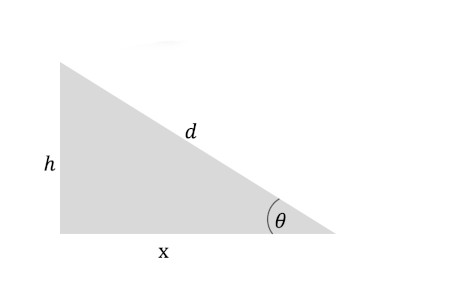# A person jogs up a hill that is at a 21% grade. He does this for a distance of 320 feet. How much...

## Question:

A person jogs up a hill that is at a 21% grade. He does this for a distance of 320 feet.

How much does his elevation increase?

There are a handful of ways to express the gradient: indirectly by the angle or directly per unit or as a percentage. In any way, the gradient represents the ratio of the side that is opposite the angel (also called the change in the vertical axis) and the side that is adjacent to the angle (i.e. the horizontal axis).

Let's use the following diagram to investigate the elevation {eq}h {/eq}.We are told that the slope has a gradient 21%. That means that {eq}\tan\theta = \frac{h}{x} = 21\% = 0.21 {/eq}.

Let's get the angle of the incline.

{eq}\begin{align*} \tan\theta &= 0.21\\ \theta &= \tan^{-1}\left (0.21\right )\\ &\approx \rm 11.9^\circ \end{align*} {/eq}

The distance up the incline is {eq}d =\rm 320\ ft {/eq}.

Using a proper trigonometric ratio that connects the distance {eq}d {/eq} and the elevation {eq}h {/eq}, the elevation can be calculated as follows:

{eq}\begin{align*} \sin\theta &= \frac{h}{d}\\ h &= d\sin\theta\\ &= \rm 320\sin 11.9^\circ\ ft\\ &\approx \rm 66\ ft \end{align*} {/eq}

The elevation for the distance given is about 66 feet.Angle of Elevation: Definition, Formula & Examples

from

Chapter 5 / Lesson 12
26K

This lesson will define an angle of elevation, and it will provide some basic skills necessary to calculate the measure of one of these types of angles or to use it to calculate another value. Two example problems are also provided.